# Cords joining

Mum needs 6 cords long 360cm long. How many does she need when they are sold at 9 meters and does not want to join it up?

Result

n =  3

#### Solution:Leave us a comment of example and its solution (i.e. if it is still somewhat unclear...):

Showing 0 comments:Be the first to comment!#### To solve this example are needed these knowledge from mathematics:

Do you want to convert length units?

## Next similar examples:

1. Street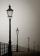Straight streets is long x = 1425 meters. At the beginning and at the end of the street are poles. The poles are spaced 25 m. How many poles are on the street?
2. FedorFedor stood in the small pool. Above the surface was a part of his body that was twice as long as part of his body underwater. What height does Fedor have, if his body part below the surface is 5 dm long?
3. School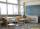27 students of 6.D class is going to a trip. They pay 9 € each. The teacher got 153 €. How many children have not paid?
4. DoctorsIn the city operates 171 doctors. The city has 128934 citizens. How many citizens are per one doctor?
5. Farm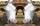Agricultural cooperative (farm) produced 14144 liters of milk for 16 days. Which exceeded the plan by 1984 liters. How many liters of milk a day had planned to produce?
6. On the farm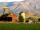The farm took 221 tons of potatoes from 17 hectares. How many tons of potatoes taken from one hectare?
7. The resultHow many times I decrease the number 1632 to get the result 24?
8. Math classificationIn 3A class are 27 students. One-third got a B in math and the rest got A. How many students received a B in math?
9. Pimps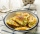There were 24 pimps in the plate. Maros ate 12, his little sister four times less. How many pimps remained in the plate?
10. Workshop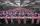In the workshop have to be produced for 5 days 2000 components. How many components must be produced in the workshop every day and how much per hour, if shift is 8 hours.
11. Number unknownAdela thought the two-digit number, she added it to its ten times and got 407. What number does she think?
12. I thinkI think a number. When I multiply it by five, and after that I subtract 477, I get the same number as if I multiplied it twice. What number do I think?
13. Roman numeralsWrite numbers written in Roman numerals as decimal.
14. DivisionWhich number in division 16 give 12 and the rest 3?
15. Dividing by five and tenNumber 5040 divide by the number 5 and by number 10: a = 5040: 5 b = 5040: 10
16. SummandOne of the summands is 145. The second is 10 more. Determine the sum of the summands.
17. What is missing (1000)What number is to add to get 1000?